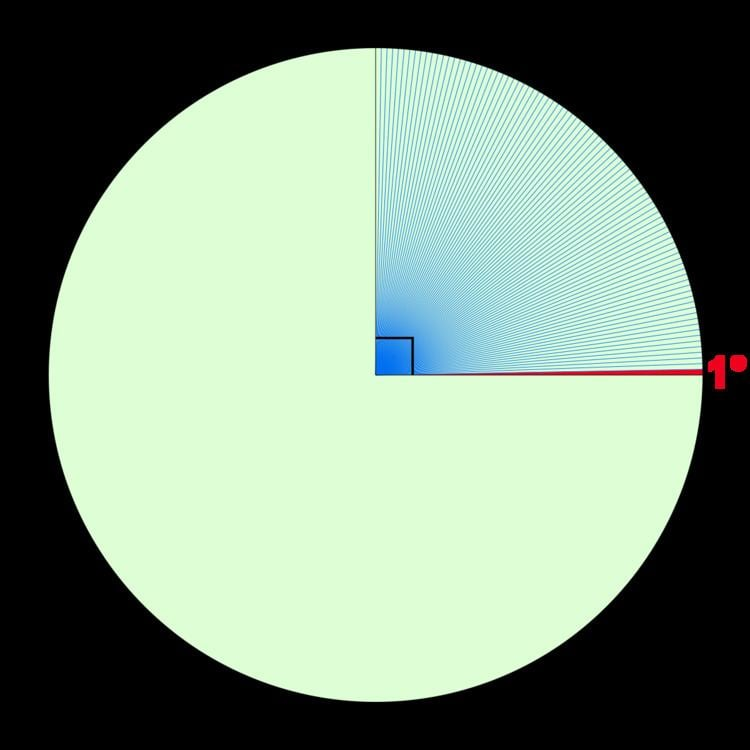# Degree (angle)

Updated on
Covid-19
 Unit system  Non-SI accepted unitSymbol  ° or degturns  1/360 turn Unit of  Angle1 ° in ...  ... is equal to ...radians  π/180 radA degree (in full, a degree of arc, arc degree, or arcdegree), usually denoted by ° (the degree symbol), is a measurement of a plane angle, defined so that a full rotation is 360 degrees.

## Contents

It is not an SI unit, as the SI unit of angular measure is the radian, but it is mentioned in the SI brochure as an accepted unit. Because a full rotation equals 2π radians, one degree is equivalent to π/180 radians.

## History

The original motivation for choosing the degree as a unit of rotations and angles is unknown. One theory states that it is related to the fact that 360 is approximately the number of days in a year. Ancient astronomers noticed that the sun, which follows through the ecliptic path over the course of the year, seems to advance in its path by approximately one degree each day. Some ancient calendars, such as the Persian calendar, used 360 days for a year. The use of a calendar with 360 days may be related to the use of sexagesimal numbers.

Another theory is that the Babylonians subdivided the circle using the angle of an equilateral triangle as the basic unit and further subdivided the latter into 60 parts following their sexagesimal numeric system. The earliest trigonometry, used by the Babylonian astronomers and their Greek successors, was based on chords of a circle. A chord of length equal to the radius made a natural base quantity. One sixtieth of this, using their standard sexagesimal divisions, was a degree.

Aristarchus of Samos and Hipparchus seem to have been among the first Greek scientists to exploit Babylonian astronomical knowledge and techniques systematically. Timocharis, Aristarchus, Aristillus, Archimedes, and Hipparchus were the first Greeks known to divide the circle in 360 degrees of 60 arc minutes. Eratosthenes used a simpler sexagesimal system dividing a circle into 60 parts.

The division of the circle into 360 parts also occurred in ancient India, as evidenced in the Rigveda:

Twelve spokes, one wheel, navels three.

Who can comprehend this?
On it are placed together
three hundred and sixty like pegs.
They shake not in the least.

Another motivation for choosing the number 360 may have been that it is readily divisible: 360 has 24 divisors, making it one of only 7 numbers such that no number less than twice as much has more divisors (sequence A072938 in the OEIS). Furthermore, it is divisible by every number from 1 to 10 except 7. This property has many useful applications, such as dividing the world into 24 time zones, each of which is nominally 15° of longitude, to correlate with the established 24-hour day convention.

Finally, it may be the case that more than one of these factors has come into play. According to that theory, the number is approximately 365 because of the apparent movement of the sun against the celestial sphere and that it was rounded to 360 for some of the mathematical reasons cited above.

## Subdivisions

For many practical purposes, a degree is a small enough angle that whole degrees provide sufficient precision. When this is not the case, as in astronomy or for geographic coordinates (latitude and longitude), degree measurements may be written using decimal degrees, with the degree symbol behind the decimals; for example, 40.1875°.

Alternatively, the traditional sexagesimal unit subdivisions can be used. One degree is divided into 60 minutes (of arc), and one minute into 60 seconds (of arc). Use of degrees-minutes-seconds is also called DMS notation. These subdivisions, also called the arcminute and arcsecond, are respectively represented by a single and double prime. For example, 40.1875° = 40° 11′ 15″, or, using quotation mark characters, 40° 11' 15". Additional precision can be provided using decimals for the arcseconds component.

The older system of thirds, fourths, etc., which continues the sexagesimal unit subdivision, was used by al-Kashi and other ancient astronomers, but is rarely used today. These subdivisions were denoted by writing the Roman numeral for the number of sixtieths in superscript: 1I for a "prime" (minute of arc), 1II for a second, 1III for a third, 1IV for a fourth, etc. Hence the modern symbols for the minute and second of arc, and the word "second" also refer to this system.

## Alternative units

In most mathematical work beyond practical geometry, angles are typically measured in radians rather than degrees. This is for a variety of reasons; for example, the trigonometric functions have simpler and more "natural" properties when their arguments are expressed in radians. These considerations outweigh the convenient divisibility of the number 360. One complete turn (360°) is equal to 2π radians, so 180° is equal to π radians, or equivalently, the degree is a mathematical constant: 1° = π180.

The turn (or revolution, full circle, full rotation, cycle) is used in technology and science. One turn is equal to 360°.

With the invention of the metric system, based on powers of ten, there was an attempt to replace degrees by decimal "degrees" called grad or gon, where the number in a right angle is equal to 100 gon with 400 gon in a full circle (1° = 109 gon). Although that idea was abandoned by Napoleon, grades continued to be used in several fields and many scientific calculators support them. Decigrades (14,000) were used with French artillery sights in World War I.

An angular mil, which is most used in military applications, has at least three specific variants, ranging from 16,400 to 16,000. It is approximately equal to one milliradian (c. 16,283). A mil measuring 16,000 of a revolution originated in the imperial Russian army, where an equilateral chord was divided into tenths to give a circle of 600 units. This may be seen on a lining plane (an early device for aiming indirect fire artillery) dating from about 1900 in the St. Petersburg Museum of Artillery.

Degree (angle) Wikipedia

Topics